This chapter introduces the strong-constraint 4-dimensional variational (SC-4DVar) method. By strong constraint, we refer to the dynamical model having no model errors. Hence, the model solution over the assimilation window is entirely determined by the model as soon as we give the initial conditions. In SC-4DVar, we solve a 4-dimensional problem by including the three space dimensions and time as the fourth dimension, using a variational approach. The method is a gradient-based minimization method but makes use of an adjoint model to calculate the gradient. The chapter covers the SC-4DVar’s standard form for estimating initial conditions and uncertain parameters. After that, it discusses a more efficient incremental formulation before presenting the state-transform variant of the method.

## 1 Standard Strong-Constraint 4DVar Method

Sasaki (1970a) introduced the concept of a strong-constraint formulation for a minimization problem when imposing a dynamical model without errors as a strong constraint. The iterative SC-4DVar method for solving the strong-constraint problem has its origin in several publications in the atmosphere and ocean modeling communities, e.g., (Lewis & Derber, 1985; Le Dimet & Talagrand, 1986; Talagrand & Courtier, 1987; Thacker, 1988; Thacker & Long, 1988) . Later , in Chap. 5, we will extend this formulation to the weak-constraint case where we allow the model to contain errors leading to the so-called weak-constraint variational inverse problem and the weak-constraint 4DVar (WC-4DVar) method.

### 1.1 Data-Assimilation Problem

We now assume the model system to include Eqs. (2.1, 2.2, and 2.5) and write it as

\begin{aligned} {\mathbf {x}}_0&= {\mathbf {x}}_0^\mathrm {f}+{\mathbf {x}}'_0, \end{aligned}
(4.1)
\begin{aligned} \boldsymbol{\theta }&= \boldsymbol{\theta }^\mathrm {f}+\boldsymbol{\theta }', \end{aligned}
(4.2)
\begin{aligned} {\mathbf {x}}_{k+1}&= {\mathbf {m}}({\mathbf {x}}_{k},\boldsymbol{\theta }), \end{aligned}
(4.3)

where there are no model errors or uncertainty in the model-state evolution, but we allow for uncertain model initial conditions and parameters. Additional constraints come from the measurements with errors

\begin{aligned} {\mathbf {d}}= {\mathbf {h}}({\mathbf {x}}) + {\mathbf {e}}. \end{aligned}
(4.4)

Thus, we wish to estimate the model’s uncertain initial conditions at the start of the assimilation window and the poorly known parameters to find a model prediction close to the measurements. At the same time, the estimated initial conditions and parameters should remain close to their first-guess values while respecting the prescribed uncertainties in both. The state vector $${\mathbf {z}}$$ contains the initial state and model parameters,

\begin{aligned} {\mathbf {z}}= \begin{pmatrix} {\mathbf {x}}_0\\ \boldsymbol{\theta }\end{pmatrix}. \end{aligned}
(4.5)

We start from the cost function in Eq. (3.9). The operator $${\mathbf {g}}({\mathbf {z}})$$ is the composite function including the model recursion from Eq. (4.3) that predicts the model solution at all time steps over the assimilation window followed by a measurement operator that maps the prediction to the measurements.

From the definition of the predicted measurements in Eq. (2.32), $${\mathbf {g}}({\mathbf {z}})={\mathbf {h}}\bigl ({\mathbf {m}}({\mathbf {z}})\bigr )$$, we can write $${\mathbf {g}}({\mathbf {z}})={\mathbf {h}}({\mathbf {x}})$$, and it is then convenient to reformulate the problem defined by the cost function in Eq. (3.9) as

SC-4DVar costfunction

\begin{aligned} \mathcal {J}({\mathbf {z}}) = \frac{1}{2} \bigl ({\mathbf {z}}-{\mathbf {z}}^\mathrm {f}\bigr )^{\mathrm {T}}\, {{\mathbf {C}}_{\textit{zz}}^{-1}}\, \bigl ({\mathbf {z}}-{\mathbf {z}}^\mathrm {f}\bigr ) + \frac{1}{2} \bigl ({\mathbf {h}}({\mathbf {x}})-{\mathbf {d}}\bigr )^{\mathrm {T}}\, {\mathbf {C}}_\textit{dd}^{-1}\,\bigl ({\mathbf {h}}({\mathbf {x}})-{\mathbf {d}}\bigr ), \end{aligned}
(4.6)

subject to the “perfect-model” constraint in Eq. (4.3), which defines the model solution $${\mathbf {x}}$$ over the assimilation window.

In SC-4DVar, we commonly refer to the state-covariance matrix

\begin{aligned} {{{\mathbf {C}}_{\textit{zz}}}} = \begin{pmatrix} {\mathbf {C}}_{x_0\!x_0} &{} {\mathbf {0}}\\ {\mathbf {0}}&{} {\mathbf {C}}_{\theta \theta } \end{pmatrix}, \end{aligned}
(4.7)

as the background-error-covariance matrix, which characterizes the error covariances of the prior initial conditions and the parameters. We specify the background-error-covariance matrix using time-independent numerical representations of prescribed relationships between variables  (Weaver et al., 2003, 2005) . We would typically not assume correlations between model parameters and the initial conditions.

### 1.2 Lagrangian Formulation

Minimizing the cost function in Eq. (4.6), subject to the additional constraint of the model Eq. (4.3), allows us to formulate a Lagrangian minimization problem with the model constraint introduced via Lagrangian multipliers $$\boldsymbol{\lambda }_k$$. Note that we already have included the prior initial condition and parameters in the first term of the cost function. By introducing the Lagrangian multipliers, we increase the number of unknowns in the optimization problem, but the formulation allows for an efficient solution method. The Lagrangian cost function for the constrained minimization problem becomes

\begin{aligned} \begin{aligned} \mathcal {L}({\mathbf {x}}_0, \dots , {\mathbf {x}}_{K+1}, \boldsymbol{\theta },\boldsymbol{\lambda }_1, \ldots , \boldsymbol{\lambda }_{K+1})&= \frac{1}{2} \bigl ({\mathbf {x}}_0-{\mathbf {x}}_0^\mathrm {f}\bigr )^{\mathrm {T}}\, {\mathbf {C}}_{x_0x_0}^{-1}\, \bigl ({\mathbf {x}}_0-{\mathbf {x}}_0^\mathrm {f}\bigr ) \\&+ \frac{1}{2} \bigl (\boldsymbol{\theta }-\boldsymbol{\theta }^\mathrm {f}\bigr )^{\mathrm {T}}\, {\mathbf {C}}_{\theta \theta }^{-1} \,\bigl (\boldsymbol{\theta }-\boldsymbol{\theta }^\mathrm {f}\bigr ) \\&+ \frac{1}{2} \bigl ({\mathbf {h}}({\mathbf {x}})-{\mathbf {d}}\bigr )^{\mathrm {T}}\,{\mathbf {C}}_\textit{dd}^{-1}\,\bigl ({\mathbf {h}}({\mathbf {x}})-{\mathbf {d}}\bigr ) \\&+ \sum _{k=0}^{K} \boldsymbol{\lambda }_{k+1}^\mathrm {T}\bigl ({\mathbf {x}}_{k+1}-{\mathbf {m}}({\mathbf {x}}_{k},\boldsymbol{\theta }) \bigr ). \end{aligned} \end{aligned}
(4.8)

The last expression in this Lagrangian introduces the Lagrange multipliers and the perfect-model constraints. In the summation, we include an extra time step for $${\mathbf {x}}_{K+1}$$ and $$\boldsymbol{\lambda }_{K+1}$$, leading to a more straightforward form of the Euler–Lagrange equations below.

We now define the gradients of the nonlinear measurement operator $${\mathbf {h}}$$ and model $${\mathbf {m}}$$ as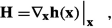(4.9)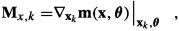(4.10)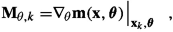(4.11)

and simplify the notation $$({\mathbf {x}}_0, \dots , {\mathbf {x}}_{K+1}, \boldsymbol{\theta }, \boldsymbol{\lambda }_1, \dots , \boldsymbol{\lambda }_{K+1})$$ by the more compact notation $$({\mathbf {x}},\boldsymbol{\theta },\boldsymbol{\lambda })$$. The time index on $${\mathbf {M}}_{\theta ,k}$$ denotes that we evaluate the gradient to $$\boldsymbol{\theta }$$ at different times $$t_k$$.

### 1.3 Explaining the Measurement Operator

We assume that $${\mathbf {H}}$$ consists of matrices $${\mathbf {H}}_k$$, each with size $$m\times n$$, with m being the number of measurements within an assimilation window, and n the size of the state vector at the time $$t_k$$. We then define one matrix $${\mathbf {H}}_k$$ for each time step $$t_k$$, that relates the predicted measurements at $$t_k$$ to the state vector at $${\mathbf {x}}_k$$. Thus,

\begin{aligned} {\mathbf {H}}= \left( \begin{array}{ccccc} {\mathbf {H}}_0 &{}\cdots &{} {\mathbf {H}}_{k} &{} \cdots &{} {\mathbf {H}}_{K+1} \\ \end{array} \right) . \end{aligned}
(4.12)

The rows in $${\mathbf {H}}$$ correspond to the m measurements distributed over the assimilation window, and $${\mathbf {H}}_k$$ corresponds to measurements available at the time step k within this window. Thus, for a time, $$t_k$$, we can have a set of measurements, and a sub-block $${\mathbf {H}}_k$$ will relate these measurements to the model state at that time. If there are no measurements at a time $$t_k$$, then $${\mathbf {H}}_k={\mathbf {0}}$$. Because of this construction, each matrix $${\mathbf {H}}_k$$ is very sparse. All rows are zero except for the rows corresponding to the measurements at time $$t_k$$ and the measurement location.

The matrix $${\mathbf {H}}$$ can take care of interpolation between the measurement location and the model discretization. In contrast, suppose the measurement is taken precisely at a model gridpoint. In that case, the corresponding row in $${\mathbf {H}}$$ will have a single element that connects the model variable to the measurement. In this manner, $${\mathbf {y}}={\mathbf {H}}{\mathbf {x}}$$ denotes the vector of all predicted measurements over the assimilation window. Likewise, $${\mathbf {y}}_k={\mathbf {H}}_k {\mathbf {x}}_k$$ is the vector $${\mathbf {y}}$$ with zeros except for the predicted measurements at time $$t_k$$ at the measurement location. Note that we have defined $${\mathbf {H}}_0={\mathbf {H}}_{K+1}={\mathbf {0}}$$. We have used this definition for $${\mathbf {H}}$$ to allow for a compact and straightforward notation in the following and at the same time allow for measurement errors correlated over time.

### 1.4 Euler–Lagrange Equations

We now find for the gradient of the Lagrangian to $${\mathbf {x}}_k$$, for $$k=1,\ldots ,K$$,

\begin{aligned} \nabla _{{\mathbf {x}}_k} \mathcal {L}({\mathbf {x}}, \boldsymbol{\theta },\boldsymbol{\lambda }) ={\mathbf {H}}_k^\mathrm {T}\, {\mathbf {C}}_\textit{dd}^{-1} \, \bigl ({\mathbf {h}}({\mathbf {x}})-{\mathbf {d}}\bigr ) + \boldsymbol{\lambda }_k-{\mathbf {M}}_{x,k}^\mathrm {T}\boldsymbol{\lambda }_{k+1}. \end{aligned}
(4.13)

The transpose of the linearized model $${\mathbf {M}}_{x,k}$$, known as the adjoint of the model, deserves some specific attention. The linearized model $${\mathbf {M}}_{x,k}$$ maps a vector from time $$t_k$$ to time $$t_{k+1}$$. Its adjoint, $${\mathbf {M}}_{x,k}^\mathrm {T}$$, does the reverse, it maps a vector from time $$t_{k+1}$$ backwards in time to $$t_k$$. Hence, Eq. (4.13) refers to a backward integration of the linearized model equations’ adjoint model.

The gradient of the Lagrangian to $${\mathbf {x}}_{K+1}$$ becomes simply

\begin{aligned} \nabla _{{\mathbf {x}}_{K+1}} \mathcal {L}({\mathbf {z}},{\mathbf {x}}, \boldsymbol{\lambda }) = \boldsymbol{\lambda }_{K+1}. \end{aligned}
(4.14)

For the initial time, we obtain the gradient of the cost function to $${\mathbf {x}}_0$$ as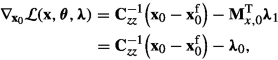(4.15)

where we have defined an additional “pseudo-variable” $$\boldsymbol{\lambda }_0$$ for convenience. The derivative of the Lagrangian to the parameters $$\boldsymbol{\theta }$$ gives

\begin{aligned} \nabla _{\boldsymbol{\theta }} \mathcal {L}({\mathbf {x}},\boldsymbol{\theta },\boldsymbol{\lambda }) = {\mathbf {C}}_{\theta \theta }^{-1} \bigl (\boldsymbol{\theta }-{\boldsymbol{\theta }}^\mathrm {f}\bigr ) -\sum _{k=0}^{K}{\mathbf {M}}^\mathrm {T}_{\theta ,k}\boldsymbol{\lambda }_{k+1}. \end{aligned}
(4.16)

Finally, the derivative of $$\mathcal {L}({\mathbf {x}},\boldsymbol{\theta },\boldsymbol{\lambda })$$ to the Lagrange multiplier $$\boldsymbol{\lambda }_k$$ returns the model equation

\begin{aligned} \nabla _{\boldsymbol{\lambda }_k} \mathcal {L}({\mathbf {x}},\boldsymbol{\theta },\boldsymbol{\lambda }) = {\mathbf {x}}_{k+1}-{\mathbf {m}}({\mathbf {x}}_k,\boldsymbol{\theta }). \end{aligned}
(4.17)

Setting the derivatives in Eqs. (4.134.17) to zero results in a coupled system of Euler–Lagrange equations consisting of a forward model

\begin{aligned} {\mathbf {x}}_0&={\mathbf {x}}_0^\mathrm {f}+ {\mathbf {C}}_{x_0x_0} \boldsymbol{\lambda }_0, \end{aligned}
(4.18)
\begin{aligned} \boldsymbol{\theta }&=\boldsymbol{\theta }^\mathrm {f}+ {\mathbf {C}}_{\theta \theta } \sum _{k=0}^{K}{\mathbf {M}}^\mathrm {T}_{\theta ,k}\boldsymbol{\lambda }_{k+1}, \end{aligned}
(4.19)
\begin{aligned} {\mathbf {x}}_{k+1}&={\mathbf {m}}({\mathbf {x}}_{k},\boldsymbol{\theta }), \end{aligned}
(4.20)

and a backward model for the adjoint variable

\begin{aligned} \boldsymbol{\lambda }_{K+1}&= 0, \end{aligned}
(4.21)
\begin{aligned} \boldsymbol{\lambda }_{k}&= {\mathbf {M}}_{x,k}^\mathrm {T}\,\boldsymbol{\lambda }_{k+1} - {\mathbf {H}}_k^\mathrm {T}\,{\mathbf {C}}_\textit{dd}^{-1}\, \bigl ({\mathbf {h}}({\mathbf {x}})-{\mathbf {d}}\bigr ). \end{aligned}
(4.22)

In this manner, we must solve a coupled two-point boundary-value problem in time. The last term of the right-hand side of Eq. (4.22) is often referred to as the weighted observational forcing  (Daley, 1991; Talagrand & Courtier, 1987) and introduces the observation information, which is brought backward from an observation time to the start of the time window.

In the standard form of SC-4DVar, we use the gradients in Eqs. (4.15) and (4.16) in a Gauss–Newton method to iteratively update the initial conditions of the forward model. Thus, starting from the first-guess solution of the model with $$\boldsymbol{\lambda }=0$$, we obtain a solution for $${\mathbf {x}}$$ from the forward model Eqs. (4.184.20). We can then use this $${\mathbf {x}}$$ to solve the adjoint model in Eqs. (4.21) and (4.22) for $$\boldsymbol{\lambda }$$. When we have an estimate of $$\boldsymbol{\lambda }$$, we can evaluate the gradients in Eqs. (4.15) and (4.16) to update the initial condition and estimate the parameters and repeat the procedure. We typically use a conjugate-gradient or a quasi-Newton method for this iterative procedure (Navon & Legler, 1987). The linearization points for the observation operator and the model, defined by the states at different times in the forward model integration, differ in each iteration, such that $${\mathbf {M}}_{x,k}^\mathrm {T}$$ and $${\mathbf {M}}_{\theta ,k}$$, and in some cases, $${\mathbf {H}}$$ will differ between iterations. In Algorithm 1, we illustrate the practical implementation of the standard SC-4DVar method.

## 2 Incremental Strong-Constraint 4DVar

In the cost function in Eq. (4.6), both the model and the measurement operators are often nonlinear. Minimizing a non-quadratic cost function can be challenging as the most efficient minimization methods, such as conjugate gradient, assume a quadratic cost function. Therefore, an approach based on the incremental Gauss–Newton formulation can lead to a more straightforward minimization problem and more efficient solvers. Thus, to practically implement SC-4DVar, one often uses the more efficient incremental form of the Gauss–Newton method from Sect. 3.5, leading to the so-called incremental  4DVar (see, e.g., Weaver et al. 2005) .

### 2.1 Incremental Formulation

Incremental 4DVar is particularly suitable for estimating initial conditions. If we want to estimate model parameters using the incremental approach, we need to update them in the outer iterations. The inner iterations perform a linearized model integration for the increments utilizing the model’s tangent-linear operator evaluated at the current model solution $${\mathbf {x}}^{i}$$ and parameters $$\boldsymbol{\theta }^{i}$$. Before addressing the parameter-estimation problem, let us focus on estimating the initial model state only. In this case, the state vector is $${\mathbf {z}}= {\mathbf {x}}_0$$, and the dynamical model with an uncertain initial condition is now

\begin{aligned} {\mathbf {x}}_0&= {\mathbf {x}}_0^\mathrm {f}+{\mathbf {x}}'_0, \end{aligned}
(4.23)
\begin{aligned} {\mathbf {x}}_{k+1}&= {\mathbf {m}}({\mathbf {x}}_{k}). \end{aligned}
(4.24)

In incremental SC-4DVar, we compute updates

\begin{aligned} {\mathbf {z}}^{i+1}={\mathbf {z}}^{i}+{\delta {\mathbf {z}}}, \end{aligned}
(4.25)

where the increments $${\delta {\mathbf {z}}}$$ are solutions that minimize the cost function in Eq. (3.24) for iteration i.

As in Sect. 4.1, $${\mathbf {g}}({\mathbf {z}})$$ is the composite function of the recursive time stepping of the model (see Eq. 2.32). Thus, we find the model solution $${\mathbf {x}}$$ over the assimilation window from Eq. (4.24) and apply the measurement operator $${\mathbf {h}}({\mathbf {x}})$$ to obtain the predicted measurements. The linearization of $${\mathbf {m}}$$ now gives the tangent-linear operator of the nonlinear model evaluated at the model solution $${\mathbf {x}}^{i}$$, from the ith outer iteration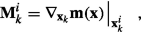(4.26)

similar to the definition in Eq. (4.10).

We will now minimize the cost function in Eq. (3.24) iteratively. We can compute the model solution’s ith increment $${\delta {\mathbf {x}}}={\delta {\mathbf {x}}}_1,..., {\delta {\mathbf {x}}}_{K+1}$$ over the assimilation window from the tangent-linear model with initial conditions

\begin{aligned} {\delta {\mathbf {x}}}_0 = {\delta {\mathbf {z}}}^{i}, \end{aligned}
(4.27)
\begin{aligned} {\delta {\mathbf {x}}}_{k+1} = {\mathbf {M}}_k^{i}{\delta {\mathbf {x}}}_k. \end{aligned}
(4.28)

For the predicted measurements of the increments, we can write $${\mathbf {H}}{\delta {\mathbf {x}}}$$, using the linearized measurement operator $${\mathbf {H}}$$ from Eq. (4.9). Additionally, for the ith iteration, we define the prior increment

\begin{aligned} \boldsymbol{\xi }^{i}={\mathbf {x}}_0^\mathrm {f}-{\mathbf {x}}_0^{i}, \end{aligned}
(4.29)

and the innovation

\begin{aligned} \boldsymbol{\eta }^{i}={\mathbf {d}}- {\mathbf {h}}({\mathbf {x}}^{i}). \end{aligned}
(4.30)

For each iteration i, the problem reduces to minimizing the cost function

Inner incremental SC-4DVar costfunction

\begin{aligned} \mathcal {J}({\delta {\mathbf {z}}}) = \frac{1}{2} \bigl ({\delta {\mathbf {z}}}- \boldsymbol{\xi }^{i}\bigr )^{\mathrm {T}}{{\mathbf {C}}_{\textit{zz}}^{-1}}\bigl ({\delta {\mathbf {z}}}- \boldsymbol{\xi }^{i}\bigr ) + \frac{1}{2} \bigl ({\mathbf {H}}^{i}{\delta {\mathbf {x}}}-\boldsymbol{\eta }^{i}\bigr )^{\mathrm {T}}\,{\mathbf {C}}_\textit{dd}^{-1}\, \bigl ({\mathbf {H}}^{i}{\delta {\mathbf {x}}}-\boldsymbol{\eta }^{i}\bigr ), \end{aligned}
(4.31)

for $${\delta {\mathbf {z}}}$$, subject to the model constraint in Eq. (4.28). Note the similarity to Eq. (3.24).

### 2.2 Lagrangian Formulation for the Inner Iterations

We again introduce Lagrange multipliers to form the extended cost function that incorporates the strong constraint of the perfect model, similar to Eq. (4.8). We also define the additional control variables $${\delta \boldsymbol{\lambda }}={\delta \boldsymbol{\lambda }}_1,...,{\delta \boldsymbol{\lambda }}_{K+1}$$. The Lagrangian cost function for the problem defined by Eqs. (4.28) and (4.31) now becomes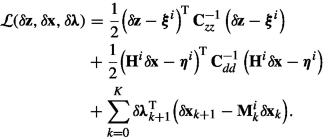(4.32)

The gradient of the Lagrangian for the incremental problem at time k becomes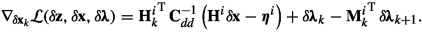(4.33)

Similarly, we have for the final time $$t_{K+1}$$,

\begin{aligned} \nabla _{{\delta {\mathbf {x}}}_K} \mathcal {L}({\delta {\mathbf {z}}}, {\delta {\mathbf {x}}}, {\delta \boldsymbol{\lambda }}) ={\delta \boldsymbol{\lambda }}_{K+1}, \end{aligned}
(4.34)

and for $$t_0$$ we find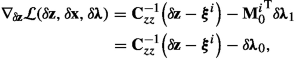(4.35)

using $${\delta {\mathbf {z}}}={\delta {\mathbf {x}}}_0$$. Finally, the derivatives of the Lagrangian to the Lagrange multipliers give the linearized forward model Eq. (4.28) in a similar way as how we arrived at Eq. (4.17) for the non-incremental 4DVar formulation.

### 2.3 Euler–Lagrange Equations for the Inner Iterations

Setting the derivatives in Eqs. (4.33), (4.34), and (4.35), and also the derivatives of the Lagrangian to $${\delta \boldsymbol{\lambda }}_k$$, all equal to zero, gives the following set of coupled Euler–Lagrange equations consisting of the linear forward model

\begin{aligned} {\delta {\mathbf {x}}}_0&= \xi ^{i}+ {{\mathbf {C}}_{\textit{zz}}}\,{\delta \boldsymbol{\lambda }}_{0}, \end{aligned}
(4.36)
\begin{aligned} {\delta {\mathbf {x}}}_{k+1} - {\mathbf {M}}_k^{i}{\delta {\mathbf {x}}}_k&= 0, \end{aligned}
(4.37)

the adjoint model with a final condition

\begin{aligned} {\delta \boldsymbol{\lambda }}_{K+1}&= 0, \end{aligned}
(4.38)
\begin{aligned} {\delta \boldsymbol{\lambda }}_k - {{\mathbf {M}}_{k}^{i}}^{\mathrm {T}}{\delta \boldsymbol{\lambda }}_{k+1}&= {{\mathbf {H}}_k^{i}}^{\mathrm {T}}{\mathbf {C}}_\textit{dd}^{-1}\bigl ({\mathbf {H}}^{i}{\delta {\mathbf {x}}}-\boldsymbol{\eta }^{i}\bigr ). \end{aligned}
(4.39)

We can now solve this coupled system efficiently using the incremental SC-4DVar (Algorithm 2).

The solution technique is similar to the one described in Sect. 4.1. We first run the nonlinear model with a first guess, $${\mathbf {z}}^\mathrm {f}$$, to compute the model state over the whole window. This model state provides the linearization points for the tangent-linear model and observation operators and defines $$\boldsymbol{\eta }^{i}$$ and $$\boldsymbol{\xi }^{i}$$. Thus, we can evaluate the full quadratic cost function in Eq. (3.24).

After that, we compute the increment $${\delta {\mathbf {z}}}$$ from the inner iterations of the linear forward model and its adjoint. We start with a first guess $${\delta {\mathbf {x}}}_0={\delta {\mathbf {z}}}$$, which we propagate forward in time with the linear model in Eq. (4.37). This solution defines the forcing field for the backward integration of the adjoint model in Eq. (4.39). The backward integration to time $$k=0$$ provides us with the gradient of the cost function in Eq. (4.35). We can then use this gradient to find a new estimate for $${\delta {\mathbf {z}}}$$ by applying methods like a conjugate gradient or a quasi-Newton technique, which feeds back into the linearized forward model in Eq. (4.37).

After minimizing the linearized cost function, we add $${\delta {\mathbf {z}}}$$ to the previous estimate $${\mathbf {x}}^{i}={\mathbf {x}}^{i+1}+{\delta {\mathbf {z}}}$$ and reevaluate $$\boldsymbol{\eta }^{i}$$ and $$\boldsymbol{\xi }^{i}$$. This update defines a new quadratic cost function and a newly updated model trajectory over the assimilation window. We then minimize this new cost function to find the next $${\delta {\mathbf {z}}}$$. Thus, there are two iterations in play, one from the Gauss–Newton process, the so-called outer loop, and one set of so-called inner iterations to solve the quadratic cost function in Eq. (3.24) for each increment $${\delta {\mathbf {z}}}$$. In the algorithm, $$\gamma$$ and $${\mathbf {B}}$$ depend on the minimization method used to solve the inner-loop problem, which can be a conjugate gradient method, BFGS, or any other minimization method.

## 3 Preconditioning in Incremental SC-4DVar

As explained in Sect. 3.4, we use a Gauss–Newton method to minimize the cost function of the increments in Eq. (4.31). We can use preconditioning for fast convergence when minimizing the cost function. The most used preconditioner is a control-variable  transform  (Bannister, 2008, Fisher et al., 2011 , Weaver et al., 2005). Remember  that we define the Gauss–Newton method’s ith estimate of the initial model state as $${\mathbf {z}}^{i}$$ and define the new iterate as $${\mathbf {z}}^{i+1}= {\mathbf {z}}^{i}+ {\delta {\mathbf {z}}}^{i}$$. The preconditioning transforms the variable $${\delta {\mathbf {z}}}$$ ($$={\delta {\mathbf {x}}}_0$$) into $${\mathbf {w}}$$ according toFootnote 1

\begin{aligned} {\delta {\mathbf {z}}}- \boldsymbol{\xi }^{i}= {\delta {\mathbf {x}}}_0 - \boldsymbol{\xi }^{i}= {\mathbf {V}}{\mathbf {w}}^{i}, \end{aligned}
(4.40)

where $${\mathbf {V}}\in \Re ^{n\times n_w}$$ with $$n_w \ge n$$. $${\mathbf {V}}$$ is of full rank and defined such that $${{\mathbf {C}}_{\textit{zz}}}={\mathbf {V}}{\mathbf {V}}^\mathrm {T}$$ and $${{\mathbf {C}}_{\textit{zz}}^{-1}}=({\mathbf {V}}^{\dagger })^\mathrm {T}{\mathbf {V}}^\dagger$$. The superscript $$\dagger$$ denotes the generalized or pseudo inverse. The matrix $${\mathbf {V}}$$ typically represents the physical laws of the system, such as geostrophic balance for oceanographic simulations. We can construct $${\mathbf {V}}$$ similarly to how we generate the background matrix in Eq. 4.7 using time-independent numerical representations of prescribed relationships between variables. For example, we might want to impose hydrostatic balance in atmospheric and ocean models, balancing the relationship between gravity and the vertical pressure gradient. The matrix $${\mathbf {V}}$$ is then constructed such that the physical variables will follow this relation.

The transformation in Eq. (4.40) will make it possible to compute the cost function’s gradients to the transformed variable $${\mathbf {w}}\in \Re ^{n_w}$$ without evaluating large matrices. With this formulation, the cost function Eq. (4.31) for the increments now becomes

Inner incremental SC-4DVar cost function with preconditioning

\begin{aligned} \mathcal {J}({\mathbf {w}}^{i}) = \frac{1}{2} {{\mathbf {w}}^{i}}^\mathrm {T}{\mathbf {w}}^{i}+ + \frac{1}{2} \bigl ({\mathbf {H}}^{i}{\delta {\mathbf {x}}}-\boldsymbol{\eta }^{i}\bigr )^{\mathrm {T}}\,{\mathbf {C}}_\textit{dd}^{-1}\, \bigl ({\mathbf {H}}^{i}{\delta {\mathbf {x}}}-\boldsymbol{\eta }^{i}\bigr ). \end{aligned}
(4.41)

It is easy to see that this control-variable transform is a form of preconditioning. If we write down the Hessian of this cost function, we find, omitting the i index for clarity,

\begin{aligned} \nabla _{{\mathbf {w}}}\nabla _{{\mathbf {w}}} \mathcal {J}({\mathbf {w}}) = {\mathbf {I}}+ {\mathbf {V}}^{\mathrm {T}} {\mathbf {G}}^{\mathrm {T}} {\mathbf {R}}^{-1} {\mathbf {G}}{\mathbf {V}}, \end{aligned}
(4.42)

while the Hessian of the original problem is

\begin{aligned} \nabla _{\delta {\mathbf {z}}}\nabla _{\delta {\mathbf {z}}} \mathcal {J}(\delta {\mathbf {z}}) = {\mathbf {C}}_{zz}^{-1} + {\mathbf {G}}^{\mathrm {T}} {\mathbf {R}}^{-1} {\mathbf {G}}. \end{aligned}
(4.43)

We immediately see that

\begin{aligned} \nabla _{{\mathbf {w}}}\nabla _{{\mathbf {w}}} \mathcal {J}({\mathbf {w}}) = {\mathbf {V}}^{\mathrm {T}} \nabla _{\delta {\mathbf {z}}}\nabla _{\delta {\mathbf {z}}} \mathcal {J}(\delta {\mathbf {z}}) {\mathbf {V}}. \end{aligned}
(4.44)

For minimizing the cost function in Eq. 4.41, it is straightforward to define a constrained minimization problem using the model definition in Eq. (4.28). We can then write the Lagrangian for this problem as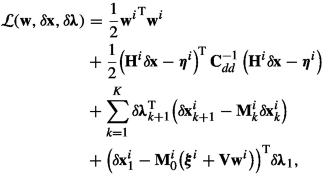(4.45)

where the last line is just the contribution corresponding to $$k=0$$ in the summation from the line above. In this transformed form, the gradient of the Lagrangian to the transformed variable $${\mathbf {w}}$$ becomes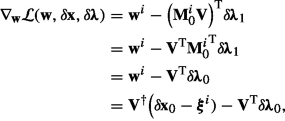(4.46)

while the gradients with respect to $${\delta {\mathbf {x}}}_k$$ and $${\delta {\mathbf {x}}}_{K+1}$$ are still the ones from Eqs. (4.33) and (4.34). Note that, by choosing $${\mathbf {x}}_0^\mathrm {f}$$ to be the initial guess for $${\mathbf {x}}_0$$, we do not need to transform from $${\delta {\mathbf {x}}}_0$$ to $${\mathbf {w}}$$, which would require computation of $${\mathbf {V}}^{\dagger }$$. Thus, the gradient of the Lagrangian to $${\mathbf {w}}$$ at time $$t=0$$ becomes

\begin{aligned} \nabla _{{\mathbf {w}}} \mathcal {L}({\mathbf {w}}, {\delta \boldsymbol{\lambda }}) = -{\mathbf {V}}^\mathrm {T}{\delta \boldsymbol{\lambda }}_{0}. \end{aligned}
(4.47)

The iteration of the above equations minimizes the cost function for the linearized model system in Eq. (4.41), and we can update the state vector, i.e., the initial conditions of the nonlinear model from

\begin{aligned} {\mathbf {z}}^{i+1}= {\mathbf {z}}^{i}+ {\delta {\mathbf {z}}}= {\mathbf {z}}^{i}+ \boldsymbol{\xi }^{i}+{\mathbf {V}}{\mathbf {w}}= {\mathbf {z}}^\mathrm {f}+ {\mathbf {V}}{\mathbf {w}}. \end{aligned}
(4.48)

We can then update $$\boldsymbol{\xi }^{i+1}$$, run the nonlinear model from $${\mathbf {z}}^{i+1}$$ to obtain the model solution, which we measure to compute $$\boldsymbol{\eta }^{i+1}$$, and start a new set of inner iterations to calculate an updated estimate of $${\mathbf {w}}$$. Typically, we only need a few outer iterations, but we run several inner-loop iterations for each outer iteration. We provide a pseudo-code in Algorithm 3, and Fig. 4.1 illustrates the process. For more information regarding the implementation of a typical 4DVar system, see, e.g., Bannister (2017) and Weaver et al. (2005).

## 4 Summary of SC-4DVar

We have seen that the SC-4DVar method solves for the minimum of a cost function. This minimum corresponds to the maximum a posteriori (MAP) probability estimate. SC-4DVar is a gradient-based method and is thus limited to weakly nonlinear problems, as for highly nonlinear problems, the descent methods are likely to get trapped in local minima. A significant obstacle for this method is the need for a tangent linear and adjoint model, which may require a considerable if not overwhelming effort in some cases. However, so-called adjoint compilers exist and have been helpful in some cases  (Marotzke et al., 1999). As one needs access to the model’s code to generate the adjoint model, SC-4DVAR is not applicable with commercial “black-box” models.

The method has formed a basis for operational weather forecasting at most international weather services. The weather community has invested a massive effort in developing, maintaining, and calibrating their SC-4DVar data-assimilation systems. A particular issue with the method is that it does not provide a simple means for computing error estimates of the analysis update or propagating updated error statistics to the next assimilation window. Thus, common in the SC-4DVar systems is the use of a stationary background matrix that one designs to represent the dynamics of the model equations (Bannister, 2008).

One of the reasons for introducing the method here, except for the historical one, is that it will serve as an essential component of an ensemble SC-4DVar configuration discussed below. Finally, we have shown that it is also possible to use SC-4DVar for pure parameter estimation.